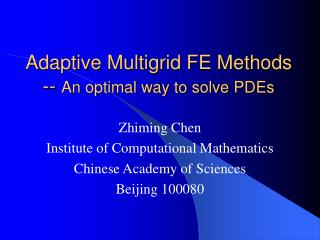DownloadDownload PresentationAdaptive Multigrid FE Methods -- An optimal way to solve PDEs

# Adaptive Multigrid FE Methods -- An optimal way to solve PDEs

Download Presentation## Adaptive Multigrid FE Methods -- An optimal way to solve PDEs

- - - - - - - - - - - - - - - - - - - - - - - - - - - E N D - - - - - - - - - - - - - - - - - - - - - - - - - - -
##### Presentation Transcript

1. Adaptive Multigrid FE Methods -- An optimal way to solve PDEs Zhiming Chen Institute of Computational Mathematics Chinese Academy of Sciences Beijing 100080

2. Adaptive Concept Refine: more nodes around singularities Coarsen: less nodes in smooth region

3. The adaptive method finds the solution of given tolerance on a self-generated mesh according to the properties of the solution (singularities,oscillations). • An “optimal mesh” is the mesh on which the error is approximately the same on each element. This motivates the error equi-distribution strategy. • The adaptive FEM based on a posteriori error estimates provides a systematic way to refine or coarsen the mesh according to the local a posteriori error estimators on each element.

4. A Linear Elliptic Problem Elliptic problem with piecewise constant coefficients: Variational problem:

5. Discrete Problem: find such that A priori error estimate:

6. A posteriori error estimate (Babuska & Miller, 1987) The error indicator where

7. Adaptive Algorithm Solve → Estimate → Refine/Coarsen Error equi-distribution strategy where tolerance, constant , number of elements in

8. Numerical Experiments where and Exact solution (Kellogg)

9. The exact solution for

10. FEM with uniform mesh 128x128 mesh: 512x512 mesh: 1024x1024 mesh: Convergence rate: A priori error analysis implies that one must introduce nodes in each space direction to bring the energy error under 0.1.

11. The surface plot of the relative error The maximum of the relative error is 0.2368.

12. The surface plot of the adaptive solution and the relative error . The maximum of the relative error is0.0188.

13. Definition Let be the sequence of FE solutions generated by the adaptive algorithm. The meshes and the associated numerical complexity are called quasi-optimal if are valid asymptotically. DOFs(k) is the number of degree of freedoms of the mesh .

14. Quasi-optimality of the estimators. The quasi-optimal decay is indicated by the dotted line of slope –1/2.

15. Gauss-Seidel Iteration Method

16. 5-point finite difference scheme

17. Multigrid V-cycle Algorithm

18. Adaptive Multigrid Method ◆ Local relaxation: Gauss-Seidel relaxation performed only on new nodes and their immediate neighboring nodes ◆ Each multigrid iteration requires only O(N) number of operations ◆ Theorem (Wu and Chen): We have ◆ Numerical Example

19. Applications • Continuous casting problem Chen, Nochetto and Schmidt (2000) • Wave scattering by periodic structure Chen and Haijun Wu (2002) • Convection diffusion problem Chen and Guanghua Ji (2003)

20. Continuous Casting problem

21. 例子：振荡铸钢速度系数 变化铸钢速度: 速度 v(t), 单元个数, 时间步长

22. t=0.05 和 t=0.07 时的网格和温度。

23. 周期结构上电磁波的散射问题

24. A Linear Convection Diffusion Problem Rotating Cylinder problem:

25. Convergence Rate Epsilon=10e-3 Epsilon=10e-5

26. Thank you !